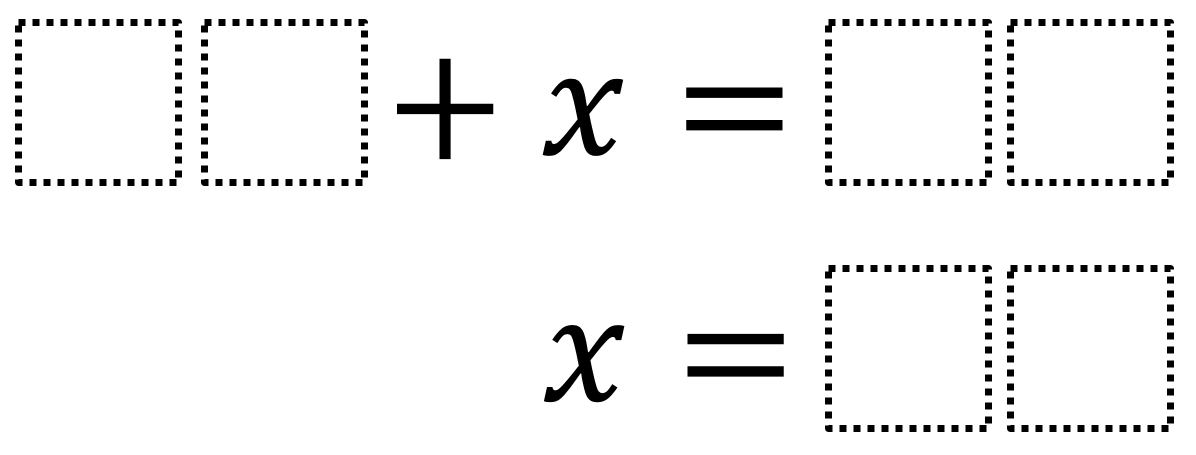Home > Grade 6 > Expressions & Equations > Solving One-Step Equations 2

# Solving One-Step Equations 2

Directions: Using the digits 1 to 9 at most one time each, fill in the boxes to make a true equation where x has the largest possible value.### Hint

How do the constants’ values affect the variable’s value?
What number has to have the highest value?
What number has to have the lowest value?

### Answer

The largest value of x is 85 when 12 + x = 97

Source: Robert Kaplinsky

## Subtracting Multi-Decimals

Directions: Use the digits 1 to 9, at most one time each, to fill in …

### 2 comments

1.The greatest value of x is 85 when 12+x =97

•Nice extension Talayla!